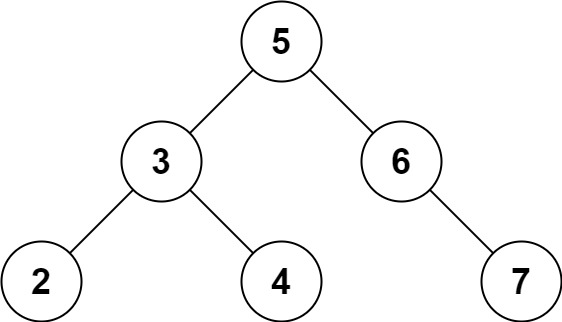# Two Sum IV Input is a BST

### 653.Two Sum IV - Input is a BST

Given the `root` of a Binary Search Tree and a target number `k`, return `true` if there exist two elements in the BST such that their sum is equal to the given target.

Example 1:```Input: root = [5,3,6,2,4,null,7], k = 9
Output: true
```

Example 2:```Input: root = [5,3,6,2,4,null,7], k = 28
Output: false
```

Example 3:

```Input: root = [2,1,3], k = 4
Output: true
```

Example 4:

```Input: root = [2,1,3], k = 1
Output: false
```

Example 5:

```Input: root = [2,1,3], k = 3
Output: true
```

Constraints:

• The number of nodes in the tree is in the range `[1, 104]`.
• `-104 <= Node.val <= 104`
• `root` is guaranteed to be a valid binary search tree.
• `-105 <= k <= 105 `

### Algorithm to solve the problemWe can do the following steps to solve the problem.We can make a vector which is will be accessible to all the functions. Let it ve 'V'.We will make a helper function in which we will perform the following operations.We will store the values of each node of the BST in inorder traversal( Inorder traversal is a way of moving to every node in a tree in the order First the Root's leftThen RootThen Root's rightWhen we are doing Inorder traversal we will store the value of the node in our vector 'V'.If the root is NULL we will simply return.In our main function, we will first call our helper function so that every value gets stored in our vector.Now we will do the same thing as we use to do in the Two Sum Problem.We will make two pointers pointing to the start and the end of the vector.We will find the sum of the values at the start and the end, and then we will have three conditions based on the sum and the target value.If our sum is greater than the target, we will decrease the value of the end pointer.If our sum is smaller than the target then we will increase the value of the start pointer.If our sum equals we simply return true.This will run until end>start . Otherwise, we return false.Our algorithm is complete, now move to the code section.The solution to the Above Question

```/**
* Definition for a binary tree node.
* struct TreeNode {
*     int val;
*     TreeNode *left;
*     TreeNode *right;
*     TreeNode() : val(0), left(nullptr), right(nullptr) {}
*     TreeNode(int x) : val(x), left(nullptr), right(nullptr) {}
*     TreeNode(int x, TreeNode *left, TreeNode *right) : val(x), left(left), right(right) {}
* };
*/
class Solution {
public: vector<int>v;
void helper(TreeNode* root){
if(root==NULL){
return;
}
helper(root->left);
v.push_back(root->val);
helper(root->right);

}

bool findTarget(TreeNode* root, int k) {
helper(root);
int start=0;
int end=v.size()-1;
while(end>start){
if(v[start]+v[end]==k){
return true;
}
else if(v[start]+v[end]>k){
end--;
}
else{
start++;
}

}
return false;
}
};```